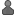1) (Message 2558) Posted 10 Jan 2018 byapohawk Post: I noticed long work units on only one system of mine. Linux, Ubuntu x86_64 16.04 LTS (for amdgpu driver), AMD Ryzen 7 1700. I have 4 tasks hanging right now. Their slots/*/error.dat is as follows: error: function Lzahbf(M,Mc) should not be called for HM stars error: function Lzahbf(M,Mc) should not be called for HM stars unexpected remnant case for K=5-6: 456132 error: function Lzahbf(M,Mc) should not be called for HM stars error: function Lzahbf(M,Mc) should not be called for HM stars unexpected remnant case for K=5-6: 490907 error: function Lzahbf(M,Mc) should not be called for HM stars error: function Lzahbf(M,Mc) should not be called for HM stars unexpected remnant case for K=5-6: 739907 error: function Lzahbf(M,Mc) should not be called for HM stars error: function Lzahbf(M,Mc) should not be called for HM stars unexpected remnant case for K=5-6: 615205 Reading through the thread i noticed, that the errrors reported in error.dat changed over time, probably being resolved in app or in input data, but the problem remains. So it is probably a layer up, something messed up with handling of the errors. I also checked what working and "hanging" tasks do. strace looks similiar, but ltrace is very different: Working task's ltrace sample (ltrace -fp PID): [pid 20453] pow(0x7f0d884383a0, 1022, 0x7f0d88491d00, 384) = 19 [pid 20453] pow(0x7f0d884383a0, 0x7f0d884363a0, 0x40c0000000000000, 333) = 279 [pid 20453] log10(0x7f0d884383a0, 0x7f0d884363a0, 0x4010000000000000, 61) = 0x7f0d88492440 [pid 20453] pow(0x7f0d884383a0, 1022, 0x7f0d88491d00, 384) = 19 [pid 20453] pow(0x7f0d884383a0, 0x7f0d884363a0, 0x40c0000000000000, 333) = 631 [pid 20453] pow(0x7f0d884383a0, 0x7f0d884363a0, 0x4000000000000000, 291) = 83 [pid 20453] pow(0x7f0d884383a0, 0x7f0d884363a0, 0x4040000000000000, 33) = 347 [pid 20453] pow(0x7f0d884383a0, 0x7f0d884363a0, 0x3fd0000000000000, 425) = 395 [pid 20453] pow(0x7f0d884383a0, 0x7f0d884363a0, 0x3fe0000000000000, 421) = 0xbff0000000000000 [pid 20453] pow(0x7f0d884383a0, 0x4002005e, 0x4002005e, 0x4002005e573c3572) = 777 [pid 20453] log10(0x7f0d884383a0, 0x7f0d884363a0, 0x4090000000000000, 377) = 0x7f0d88492440 [pid 20453] pow(0x7f0d884383a0, 1022, 0x7f0d88491d00, 384) = 19 [pid 20453] pow(0x7f0d884383a0, 0x7f0d884363a0, 0x40c0000000000000, 333) = 279 [pid 20453] log10(0x7f0d884383a0, 0x7f0d884363a0, 0x4010000000000000, 61) = 0x7f0d88492440 [pid 20453] pow(0x7f0d884383a0, 1022, 0x7f0d88491d00, 384) = 19 [pid 20453] pow(0x7f0d884383a0, 0x7f0d884363a0, 0x40c0000000000000, 333) = 631 [pid 20454] gettimeofday(0x888cde30, 0) = 0 [pid 20454] gettimeofday(0x888cde30, 0) = 0 [pid 20454] usleep(100000 [pid 20453] pow(0x7f0d884383a0, 0x7f0d884363a0, 0x4000000000000000, 291) = 83 [pid 20453] pow(0x7f0d884383a0, 0x7f0d884363a0, 0x4040000000000000, 33) = 725 [pid 20453] pow(0x7f0d884383a0, 0x7f0d884363a0, 0x3f40000000000000, 553) = 333 [pid 20453] pow(0x7f0d884383a0, 0x7f0d884363a0, 0x40e0000000000000, 483) = 421 [pid 20453] pow(0x7f0d884383a0, 0x7f0d884363a0, 0x4180000000000000, 39) = 373 [pid 20453] pow(0x7f0d884383a0, 0x7f0d884363a0, 0x40b0000000000000, 527) = 903 [pid 20453] pow(0x7f0d884383a0, 0x7f0d884363a0, 0x40f0000000000000, 319) = 725 [pid 20453] log10(0x7f0d884383a0, 0x7f0d884363a0, 0x3f40000000000000, 553) = 0x7f0d88492440 [pid 20453] pow(0x7f0d884383a0, 1022, 0x7f0d88491d00, 384) = 19 [pid 20453] pow(0x7f0d884383a0, 0x7f0d884363a0, 0x40c0000000000000, 333) = 431 [pid 20453] pow(0x7f0d884383a0, 0x7f0d884363a0, 0x4000000000000000, 139) = 61 [pid 20453] log10(0x7f0d884383a0, 0x7f0d884363a0, 0x3ff0000000000000, 605) = 0x7f0d88492440 [pid 20453] pow(0x7f0d884383a0, 1022, 0x7f0d88491d00, 384) = 19 [pid 20453] pow(0x7f0d884383a0, 0x7f0d884363a0, 0x40c0000000000000, 333) = 279 and so on... "hanging" task's ltrace sample (ltrace -fp PID): [pid 13889] free(0x1a4e560) = [pid 13889] free(0x1a4e530) = [pid 13889] free(0x1a4e500) = [pid 13889] free(0x1a4e4d0) = [pid 13889] free(0x1a4e4a0) = [pid 13889] free(0x1a4e470) = [pid 13889] __isnan(0xffffffff, 0x7fc692dddb30, 0x1a4e490, 0) = 1 [pid 13889] malloc(40) = 0x1a4e470 [pid 13889] malloc(40) = 0x1a4e4a0 [pid 13889] malloc(40) = 0x1a4e4d0 [pid 13889] malloc(40) = 0x1a4e500 [pid 13889] malloc(40) = 0x1a4e530 [pid 13889] malloc(40) = 0x1a4e560 [pid 13889] free(0x1a4e560) = [pid 13889] free(0x1a4e530) = [pid 13889] free(0x1a4e500) = [pid 13889] free(0x1a4e4d0) = [pid 13889] free(0x1a4e4a0) = [pid 13889] free(0x1a4e470) = [pid 13889] __isnan(0xffffffff, 0x7fc692dddb30, 0x1a4e490, 0) = 1 [pid 13889] malloc(40) = 0x1a4e470 [pid 13889] malloc(40) = 0x1a4e4a0 [pid 13889] malloc(40) = 0x1a4e4d0 [pid 13889] malloc(40) = 0x1a4e500 [pid 13889] malloc(40) = 0x1a4e530 [pid 13889] malloc(40) = 0x1a4e560 [pid 13889] free(0x1a4e560) = [pid 13889] free(0x1a4e530) = [pid 13889] free(0x1a4e500) = [pid 13889] free(0x1a4e4d0) = [pid 13889] free(0x1a4e4a0) = [pid 13889] free(0x1a4e470) = [pid 13889] __isnan(0xffffffff, 0x7fc692dddb30, 0x1a4e490, 0) = 1 Looks like infinite loop to me. "hanging" task's gdb backtrace taken at random: (gdb) bt #0 get_T (L=L@entry=651959.17475758004, R=R@entry=1.60374190786905) at singl.c:4688 #1 0x0000000000418274 in dorbdt (t=, y=y@entry=0x1a4e560, dydx=dydx@entry=0x1a4e4d0, KTa=KTa@entry=0, KTb=KTb@entry=2.9179459219507452e-10, Ma=Ma@entry=1.4399999999999999, Mb=Mb@entry=24.12289019752216, dMa=dMa@entry=nan(0x8000000000000), dMb=dMb@entry=0.55262531449484709, Ia=Ia@entry=-nan(0x8000000000000), Ib=Ib@entry=4.6753715902533024, Ra=Ra@entry=1.60374190786905, Rb=Rb@entry=1.6037419078694282, Rca=Rca@entry=inf, Rcb=Rcb@entry=0, wcrit_a=wcrit_a@entry=0, wcrit_b=wcrit_b@entry=0, La=La@entry=651959.17475758004, Lb=Lb@entry=651959.17475832114, Ka=Ka@entry=-978270896, Kb=Kb@entry=7, magb_a=magb_a@entry=0, magb_b=magb_b@entry=0) at binary.cpp:7817 #2 0x000000000043244c in rkck1 (y=0x1a4f190, dydx=0x1a4f1c0, n=4, x=3.4806341581987525, h=, yout=0x1a4f220, yerr=0x1a4f1f0, derivs1=0x4181d0 , KTa=0, KTb=2.9179459219507452e-10, Ma=1.4399999999999999, Mb=24.12289019752216, dMa=nan(0x8000000000000), dMb=0.55262531449484709, Ia=-nan(0x8000000000000), Ib=4.6753715902533024, Ra=1.60374190786905, Rb=1.6037419078694282, Rca=inf, Rcb=0, wcrit_a=0, wcrit_b=0, La=651959.17475758004, Lb=651959.17475832114, Ka=-978270896, Kb=7, magb_a=0, magb_b=0) at binary.cpp:8427 #3 0x000000000043398f in rkqs1 (y=y@entry=0x1a4f190, dydx=dydx@entry=0x1a4f1c0, n=n@entry=4, x=x@entry=0x7ffdc5b0b9b0, htry=0, eps=nan(0x8000000000000), eps@entry=0.001, yscal=yscal@entry=0x1a4f160, hdid=hdid@entry=0x7ffdc5b0b9d0, hnext=hnext@entry=0x7ffdc5b0b9c0, derivs1=derivs1@entry=0x4181d0 , KTa=KTa@entry=0, KTb=KTb@entry=2.9179459219507452e-10, Ma=Ma@entry=1.4399999999999999, Mb=Mb@entry=24.12289019752216, dMa=dMa@entry=nan(0x8000000000000), dMb=dMb@entry=0.55262531449484709, Ia=Ia@entry=-nan(0x8000000000000), Ib=Ib@entry=4.6753715902533024, Ra=Ra@entry=1.60374190786905, Rb=Rb@entry=1.6037419078694282, Rca=Rca@entry=inf, Rcb=Rcb@entry=0, wcrit_a=wcrit_a@entry=0, wcrit_b=wcrit_b@entry=0, La=La@entry=651959.17475758004, Lb=Lb@entry=651959.17475832114, Ka=Ka@entry=-978270896, Kb=Kb@entry=7, magb_a=magb_a@entry=0, magb_b=magb_b@entry=0) at binary.cpp:8365 #4 0x000000000042f0ff in odeint1 (ystart=ystart@entry=0x1a4f130, nvar=nvar@entry=4, x1=x1@entry=3.4806341581987525, x2=x2@entry=3.4806531067030479, eps=eps@entry=0.001, h1=0.15274083617124923, hmin=hmin@entry=0, nok=nok@entry=0x7ffdc5b0bc50, nbad=nbad@entry=0x7ffdc5b0bc60, derivs1=derivs1@entry=0x4181d0 , rkqs1=rkqs1@entry=0x433760 , KTa=0, KTb=2.9179459219507452e-10, Ma=Ma@entry=1.4399999999999999, Mb=Mb@entry=24.12289019752216, dMa=dMa@entry=nan(0x8000000000000), dMb=dMb@entry=0.55262531449484709, Ia=Ia@entry=-nan(0x8000000000000), Ib=Ib@entry=4.6753715902533024, Ra=Ra@entry=1.60374190786905, Rb=Rb@entry=1.6037419078694282, Rca=Rca@entry=inf, Rcb=Rcb@entry=0, wcrit_a=wcrit_a@entry=0, wcrit_b=wcrit_b@entry=0, La=La@entry=651959.17475758004, Lb=Lb@entry=651959.17475832114, Ka=Ka@entry=-978270896, Kb=Kb@entry=7, magb_a=magb_a@entry=0, magb_b=magb_b@entry=0) at binary.cpp:8326 #5 0x0000000000430c93 in orb_change (t1=t1@entry=3.4806341581987525, t2=t2@entry=3.4806531067030479, a=a@entry=0x7ffdc5b0c400, e=e@entry=0x7ffdc5b0c410, wa=wa@entry=0x7ffdc5b0c420, wb=wb@entry=0x7ffdc5b0c430, tvira=0, tvirb=3.7897020774656023e-05, Ma=1.4399999999999999, Mb=24.12289019752216, M0a=1.4399999999999999, M0b=24.12289019752216, Mzamsa=147.78874082664947, Mzamsb=125.59129163845198, dMwinda=dMwinda@entry=nan(0x8000000000000), dMwindb=dMwindb@entry=0.55262531449484709, Mca=0, Mcb=0, Ra=1.60374190786905, Rb=1.6037419078694282, Raold=Raold@entry=nan(0x8000000000000), Rbold=Rbold@entry=1.6037347409964342, La=651959.17475758004, Lb=651959.17475832114, Ka=-978270896, Kb=7, Kaold=Kaold@entry=1, Kbold=Kbold@entry=7, mt=mt@entry=0, ce=ce@entry=0, mttype=0, Iaold=Iaold@entry=0x7ffdc5b0c440, Ibold=Ibold@entry=0x7ffdc5b0c450, KTa=KTa@entry=0x7ffdc5b0c590, KTb=KTb@entry=0x7ffdc5b0c598, dMmta=0, dMmtb=0, Mdisa=0, Mdisb=0, darwin=darwin@entry=0x7ffdc5b0c2e4) at binary.cpp:7750 #6 0x000000000040f58c in main (argc=, argv=) at binary.cpp:1899 Those NAN are quite high in backtrace, but i don't know whether they should be there or not.